Next: Poisson's equation Up: Electrostatics Previous: Boundary conditions on the

## Capacitors

We can store electrical charge on the surface of a conductor. However, electric fields will be generated immediately above this surface. The conductor can only successfully store charge if it is electrically insulated from its surroundings. Air is a very good insulator. Unfortunately, air ceases to be an insulator when the electric field-strength through it exceeds some critical value which is aboutvolts per meter. This phenomenon, which is called break-down, is associated with the formation of sparks. The most well-known example of the break-down of air is during a lightning strike. Clearly, a good charge storing device is one which holds a large amount of charge but only generates small electric fields. Such a device is called a capacitor.

Consider two thin, parallel, conducting plates of cross-sectional areawhich are separated by a small distance(i.e.,). Suppose that each plate carries an equal and opposite charge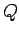. We expect this charge to spread evenly over the plates to give an effective sheet charge densityon each plate. Suppose that the upper plate carries a positive charge and that the lower plate carries a negative charge. According to Eqs. (624) and (625), the field generated by the upper plate is normal to the plate and of magnitude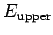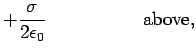(638)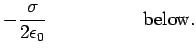(639)

Likewise, the field generated by the lower plate is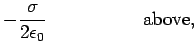(640)(641)

Note that we are neglecting any leakage'' of the field at the edges of the plates. This is reasonable if the plates are closely spaced. The total field is the sum of the two fields generated by the upper and lower plates. Thus, the net field is normal to the plates, and of magnitude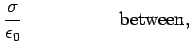(642)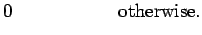(643)

Since the electric field is uniform, the potential difference between the plates is simply(644)

It is conventional to measure the capacity of a conductor, or set of conductors, to store charge, but generate small electric fields, in terms of a parameter called the capacitance. This is usually denoted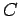. The capacitance of a charge storing device is simply the ratio of the charge stored to the potential difference generated by the charge. Thus,(645)

Clearly, a good charge storing device has a high capacitance. Incidentally, capacitance is measured in coulombs per volt, or farads. This is a rather unwieldy unit, since good capacitors typically have capacitances which are only about one millionth of a farad. For a parallel plate capacitor, it is clear that(646)

Note that the capacitance only depends on geometric quantities, such as the area and spacing of the plates. This is a consequence of the superposability of electric fields. If we double the charge on conductors then we double the electric fields generated around them, and we, therefore, double the potential difference between the conductors. Thus, the potential difference between the conductors is always directly proportional to the charge carried: the constant of proportionality (the inverse of the capacitance) can only depend on geometry.

Suppose that the charge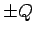on each plate is built up gradually by transferring small amounts of charge from one plate to another. If the instantaneous charge on the plates is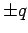, and an infinitesimal amount of positive charge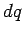is transferred from the negatively charged plate to the positively charge plate, then the work done is, whereis the instantaneous voltage difference between the plates. Note that the voltage difference is such that it opposes any increase in the charge on either plate. The total work done in charging the capacitor is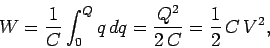(647)

where use has been made of Eq. (645). The energy stored in the capacitor is the same as the work required to charge up the capacitor. Thus,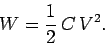(648)

This is a general result which holds for all types of capacitor.

The energy of a charged parallel plate capacitor is actually stored in the electric field between the plates. This field is of approximately constant magnitude, and occupies a region of volume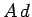. Thus, given the energy density of an electric field,, the energy stored in the electric field is(649)

where use has been made of Eq. (646). Note that Eqs. (647) and (649) agree. We all know that if we connect a capacitor across the terminals of a battery then a transient current flows as the capacitor charges up. The capacitor can then be placed to one side, and, some time later, the stored charge can be used: for instance, to transiently light a bulb in an electrical circuit. What is interesting here is that the energy stored in the capacitor is stored as an electric field, so we can think of a capacitor as a device which either stores energy in, or extracts energy from, an electric field.

The idea, which we discussed earlier, that an electric field exerts a negative pressure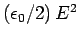on conductors immediately suggests that the two plates in a parallel plate capacitor attract one another with a mutual force(650)

It is not necessary to have two oppositely charged conductors in order to make a capacitor. Consider an isolated sphere of radiuswhich carries a charge. The radial electric field generated outside the sphere is given by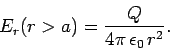(651)

The potential difference between the sphere and infinity, or, more realistically, some large, relatively distant reservoir of charge such as the Earth, is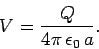(652)

Thus, the capacitance of the sphere is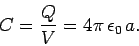(653)

The energy of a sphere when it carries a chargeis again given by. It can easily be demonstrated that this is really the energy contained in the electric field around the sphere.

Suppose that we have two spheres of radiiand, respectively, which are connected by an electric wire. The wire allows charge to move back and forth between the spheres until they reach the same potential (with respect to infinity). Letbe the charge on the first sphere and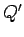the charge on the second sphere. Of course, the total charge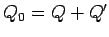carried by the two spheres is a conserved quantity. It follows from Eq. (652) that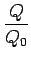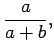(654)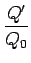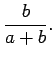(655)

Note that if one sphere is much smaller than the other one, e.g.,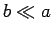, then the large sphere grabs most of the charge: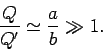(656)

The ratio of the electric fields generated just above the surfaces of the two spheres follows from Eqs. (651) and (656):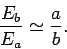(657)

If, then the field just above the smaller sphere is far larger than that above the larger sphere. Equation (657) is a simple example of a far more general rule. The electric field above some point on the surface of a conductor is inversely proportional to the local radius of curvature of the surface.

It is clear that if we wish to store significant amounts of charge on a conductor then the surface of the conductor must be made as smooth as possible. Any sharp spikes on the surface will inevitably have comparatively small radii of curvature. Intense local electric fields are generated in these regions. These can easily exceed the critical field for the break-down of air, leading to sparking and the eventual loss of the charge on the conductor. Sparking can also be very destructive because the associated electric currents flow through very localized regions giving rise to intense ohmic heating.

As a final example, consider two co-axial conducting cylinders of radiiand, where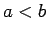. Suppose that the charge per unit length carried by the outer and inner cylinders is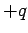and, respectively. We can safely assume that, by symmetry (adopting standard cylindrical polar coordinates). Let us apply Gauss' law to a cylinder of radius, co-axial with the conductors, and of length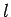. For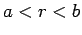, we find that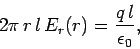(658)

so(659)

for. It is fairly obvious that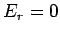ifis not in the rangeto. The potential difference between the inner and outer cylinders is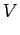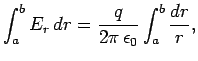(660)

so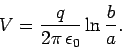(661)

Thus, the capacitance per unit length of the two cylinders is(662)Next: Poisson's equation Up: Electrostatics Previous: Boundary conditions on the
Richard Fitzpatrick 2006-02-02